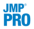Publication date: 11/29/2021

## Score Options

Score Options provides the following selections that deal with scores:

Show Interesting Rows Only

In the Discriminant Scores report, shows only rows that are misclassified and those with predicted probability between 0.05 and 0.95.

Show Classification Counts

Shows or hides the confusion matrices and confusion rates matrices in the Score Summaries report. A confusion matrix is a two-way classification of actual and predicted responses. A confusion rates matrix is equal to the confusion matrix, with the numbers divided by the row totals. By default, the Score Summaries report shows a confusion matrix and a confusion rates matrix for each level of the categorical X. If you are using JMP Pro with validation, a matrix is given for each set of observations. If you are using JMP with excluded rows, these rows are considered the validation set and a separate Validation matrix is given. See Validation in JMP and JMP Pro.

Show Distances to Each Group

Shows or hides a report that contains each observation’s squared Mahalanobis distance to each group mean.

Show Probabilities to Each Group

Shows or hides a report that contains the probability that an observation belongs to each of the groups defined by the categorical X.

ROC Curve

Shows or hides a Receiver Operating Characteristic (ROC) curve to the Score Summaries report. For more information about the ROC Curve, see ROC Curve in Predictive and Specialized Modeling.

Select Misclassified Rows

Selects the misclassified rows in the data table and in report windows that display a listing by row.

Select Uncertain Rows

Selects rows with uncertain classifications in the data table and in report windows that display a listing by row. An uncertain row is one whose probability of group membership for any group is neither close to 0 nor close to 1.

When you select this option, a window opens where you can specify the range of predicted probabilities that reflect uncertainty. By default, any row whose probability differs from 0 or 1 by more than 0.1 is defined to be uncertain. Therefore, the default selects rows with probabilities between 0.1 and 0.9.

Save Formulas

Saves distance, probability, and predicted membership formulas to the data table. See Saved Formulas.

The distance formulas are SqDist0 and SqDist[<level>], where <level> represents a level of X. The distance formulas produce intermediate values connected with the Mahalanobis distance calculations.

The probability formulas are Prob[<level>], where <level> represents a level of X. Each probability column gives the posterior probability of an observation’s membership in that level of X. The Response Probability column property is saved to each probability column. For more information about the Response Probability column property, see Using JMP.

The predicted membership formula is Pred <X> and contains the “most likely level” classification rule.

The Wide Linear method also saves a Discrim Data Matrix column containing the vector of covariates and a Discrim Prin Comp formula. See Wide Linear Discriminant Method.

Note: For any method other than Wide Linear, when you Save Formulas, a RowEdit Prob script is saved to the data table. This script selects uncertain rows in the data table. The script defines any row whose probability differs from 0 or 1 by more than 0.1 as uncertain. The script also opens a Row Editor window that enables you to examine the uncertain rows. If you fit a new model (other than Wide Linear) and select Save Formulas, any existing RowEdit Prob script is replaced with a script that applies to the new fit.

Make Scoring Script

(Available only in JMP Standard.) Creates a script that constructs the formula columns saved by the Save Formulas option. You can save this script and use it, perhaps with other data tables, to create the formula columns that calculate membership probabilities and predict group membership.Publish Probability Formulas

(Available only in JMP Pro.) Creates probability formulas and saves them as formula column scripts in the Formula Depot platform. If a Formula Depot report is not open, this option creates a Formula Depot report. See Formula Depot in Predictive and Specialized Modeling.